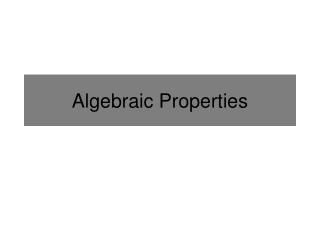# Algebraic Properties - PowerPoint PPT PresentationDownload PresentationAlgebraic Properties

Algebraic PropertiesDownload Presentation## Algebraic Properties

- - - - - - - - - - - - - - - - - - - - - - - - - - - E N D - - - - - - - - - - - - - - - - - - - - - - - - - - -
##### Presentation Transcript

1. Algebraic Properties

2. Commutative Properties Commutative Property of Addition: When adding two or more numbers together, order is not important Commutative Property of Multiplication: When multiplying two or more numbers together, order is not important Are the two expressions equivalent? There are no Commutative Properties for Subtraction and Division.

3. Associative Properties Associative Property of Addition: When adding more than two numbers the grouping of the numbers does not change the sum. Associative Property of Multiplication: When multiplying more than two numbers the grouping of the numbers does not change the product. Are the two expressions equivalent? Are there Associative Properties for Subtraction and Division?

4. Identity Properties What number can you multiply a number by to not change its value? Identity Property of Addition: The sum of any expression and zero is itself: Identity Property of Multiplication: The product of any expression and one is itself: What number can you add to a number to not change its value? There are no Identity Properties for Subtraction and Division.

5. Inverse Properties What number can you multiply a number by to get 1 (the multiplicative identity)? Additive Inverse Property: The additive inverse of any number “a” is the opposite of “a.” The sum of a number and its additive inverse is 0. Multiplicative Inverse Property: The multiplicative inverse of any number “a” is the reciprocal of “a.” The product of a number and its multiplicative inverse is 1. What number can you add to a number to get 0 (the additive identity)? There are no Inverse Properties for Subtraction and Division.

6. Justifying with Algebraic Properties For the work below, justify each step by naming the property that was used or other valid reasoning. Given Commutative Property of Addition 8 = 3 + 5 Inverse Property of Addition Identity Property of Addition

7. Is it Correct? Examine the work below. Is the work valid? If so, justify your conclusion by naming the algebraic properties. If not, explain which statement (or statements) is incorrect. The work is invalid. The circled step incorrectly applied the Associative Property. There is no Associative Property of subtraction. Only addition and multiplication.